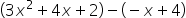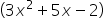Maths-
General
Easy

Question

# Simplify. Write each answer in its standard form.## The correct answer is: • A polynomial is in standard form when all its terms are arranged by decreasing order of degree.

### We have been given a function in the question. We will have to simplify it and further write the answer in its standard form. Step 1 of 1:We know that in polynomial we add/subtract like termsSo,Now, We know that the terms are written in descending order of their degree.So, In the standard formThe given polynomial will be#### With Turito Foundation.#### Get an Expert Advice From Turito.